# WindowCertification Kubus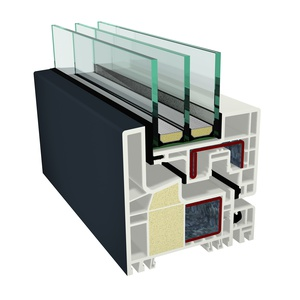Component id: 1249wi04 GEALAN Fenster-Systeme GmbH Window System PVC 1.00 W/(m2 K) phB SWISSPACER Ultimate Warm, temperate The temperaturefactor is not acheived at the threshold.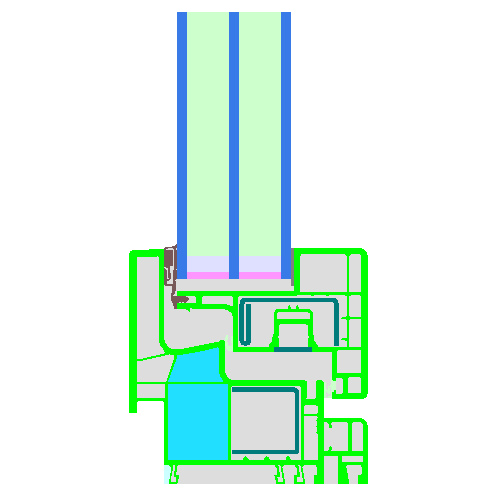Frame cut Frame width
bf/mm
Frame U value
Uf/(W/(m2 K))
Glass edge Ψ value
Ψg/(W/(m K))
Temperature factor
fRsi = 0,25 m2K/W
Threshold 74 2.50 0.024 0.56
Bottom 100 1.05 0.022 0.69
Transom 2 casements 154 1.42 0.023 0.65
Transom Fixed 100 1.36 0.023 0.66
Top Fixed 100 0.98 0.023 0.70
Bottom Fixed 100 1.05 0.022 0.69
Lateral Fixed 100 0.98 0.023 0.70
Transom 1 casement 100 1.36 0.023 0.66
Lateral 100 0.98 0.023 0.70
Flying Mullion 100 1.36 0.023 0.66
Mullion Fixed 100 1.36 0.023 0.66
Mullion 2 casements 154 1.42 0.023 0.65
Mullion 1 casement 100 1.36 0.023 0.66
Top 100 0.98 0.023 0.70

### Please select preferred window-layout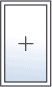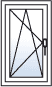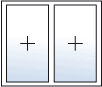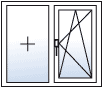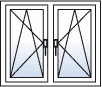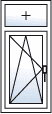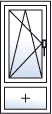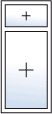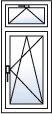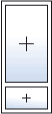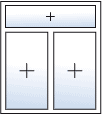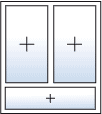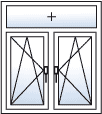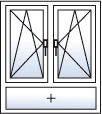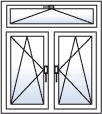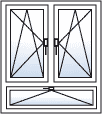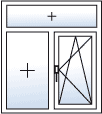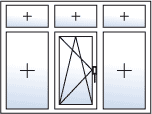### U-Value Calculation

 b1 =  m b2 =  m b3 =  m htop =  m hbottom =  mGlazing Standard (Ug = 0.7) arcon N solar (Ug = 0.6) arcon N solar (Ug = 0.69) arcon N solar (Ug = 0.64) arcon N solar (Ug = 0.77) arcon N solar (Ug = 0.67) arcon N solar (Ug = 0.75) ClimaGuard nrG (Ug = 0.6) ClimaGuard nrG (Ug = 0.69) ClimaGuard nrG (Ug = 0.75) ClimaGuard nrG (Ug = 0.64) ClimaGuard Premium (Ug = 0.49) ClimaGuard Premium (Ug = 0.64) ClimaGuard Premium2 (Ug = 0.64) ClimaGuard Premium2 (Ug = 0.49) iplus 3 CE (Ug = 0.49) iplus 3 CE (Ug = 0.56) iplus 3 CLS und CLST (Ug = 0.6) iplus 3 CLS und CLST (Ug = 0.67) iplus 3 E (Ug = 0.58) iplus 3 E (Ug = 0.64) iplus 3 E (Ug = 0.72) iplus 3 LS und LST (Ug = 0.75) iplus 3 LS und LST (Ug = 0.69) iplus 3 LS und LST helles float (Ug = 0.75) iplus 3 LS und LST helles float (Ug = 0.69) iplus Advanced 1.0 (Ug = 0.51) iplus Advanced 1.0 (Ug = 0.59) iplus Top 1.1 (Ug = 0.58) iplus Top 1.1 (Ug = 0.49) iplus Top 1.1 (Ug = 0.64) iplus Top 1.1 (Ug = 0.61) iplus Top 1.1 (Ug = 0.56) iplus Top 1.1T (Ug = 0.49) iplus Top 1.1T (Ug = 0.58) iplus Top 1.1T (Ug = 0.56) iplus Top 1.1T (Ug = 0.61) iplus Top 1.1T (Ug = 0.64) Passiv-Therm (Ug = 0.6) Planibel Tri (Ug = 0.73) Planibel Tri (Ug = 0.67) Planibel Tri (Ug = 0.75) Planibel Tri (Ug = 0.62) Planibel Tri (Ug = 0.65) Planibel Tri (Ug = 0.58) RX WARM (Ug = 0.56) RX WARM (Ug = 0.64) RX WARM (Ug = 0.72) RX WARM (Ug = 0.49) RX WARM (Ug = 0.66) RX WARM (Ug = 0.58) Solar-Therm (Ug = 0.71) Super-Therm (Ug = 0.79) TOP Premium 0.6 (Ug = 0.58) TOP Premium E 0.6 (Ug = 0.58) TOP Solar 0.7 (Ug = 0.69) Ultra-Therm (Ug = 0.6) VITAL 0.6 (Ug = 0.6) VITAL 0.6 (Ug = 0.66) VITAL 0.6 (Ug = 0.55) VITAL 0.7 (Ug = 0.69) VITAL 0.7 (Ug = 0.64) VITAL 0.7 (Ug = 0.75) SGG CLIMATOP ECLAZ ONE & ONE II (Ug = 0.67) EAGON SUPER VIG (Ug = 0.51) ClimaGuard Premium2 (Ug = 0.53) ClimaGuard Premium2 (Ug = 0.58) SGG CLIMATOP PLANITHERM ONE & ONE II (Ug = 0.6) SGG CLIMATOP PLANITHERM XN & XN II (Ug = 0.71) SGG CLIMATOP PLANITHERM LUX & LUX II (Ug = 0.79) SGG CLIMATOP PLANITHERM LUX & LUX II (Ug = 0.63) SGG CLIMATOP PLANITHERM LUX & LUX II (Ug = 0.72) SGG CLIMATOP PLANITHERM LUX & LUX II (Ug = 0.66) SGG CLIMATOP PLANITHERM XN & XN II (Ug = 0.64) EAGON SUPER VIG Plus_(air) (Ug = 0.47) SGG CLIMATOP PLANITHERM XN & XN II (Ug = 0.58) EAGON SUPER VIG Plus_Ar (Ug = 0.34) SGG CLIMATOP PLANITHERM XN & XN II (Ug = 0.55) EAGON SUPER VIG Plus_Kr (Ug = 0.33) SGG CLIMATOP ECLAZ & ECLAZ II (Ug = 0.55) SGG CLIMATOP PLANITHERM ONE & ONE II (Ug = 0.54) SGG CLIMATOP PLANITHERM ONE & ONE II (Ug = 0.5) SGG CLIMATOP ECLAZ & ECLAZ II (Ug = 0.71) iplus Advanced 1.0 (Ug = 0.53) iplus Advanced 1.0 (Ug = 0.56) iplus Advanced 1.0 (Ug = 0.44) SGG CLIMATOP ECLAZ & ECLAZ II (Ug = 0.64) SGG CLIMATOP ECLAZ & ECLAZ II (Ug = 0.58) SGG CLIMATOP ECLAZ ONE & ONE II (Ug = 0.5) SGG CLIMATOP ECLAZ ONE & ONE II (Ug = 0.54) SGG CLIMATOP ECLAZ ONE & ONE II (Ug = 0.6) iplus 3 (Ug = 0.58) iplus 3 (Ug = 0.72) iplus 3 (Ug = 0.64) iplus 3C (Ug = 0.56) iplus 3C (Ug = 0.49) iplus 3CLS (Ug = 0.6) iplus 3LS (Ug = 0.74) iplus 3CLS (Ug = 0.67) iplus 3LS (Ug = 0.69) Ug  W/(m2 K) G-Value UW = ? W/(m2 K)

### Installationsituation

UW, installed = ? W/(m2 K)

### Cost Calculation

Orientation :

Please choose orientation of the window
to get the PHPP-standard values for solar radiation

kKh/a

kWh/(m2a)

Recommended Value for Reduction Factor is 0.9
Shading Factor should be between 0.1 and 1. For standard situations look in ISO EN 13790

€/kWh

%

Years

Present Value(energy) = ? €
Present Value(total) = ? €
Back to list